Work and Kinetic Energy

# Power

### Learning Objectives

By the end of this section, you will be able to:

• Relate the work done during a time interval to the power delivered
• Find the power expended by a force acting on a moving body

The concept of work involves force and displacement; the work-energy theorem relates the net work done on a body to the difference in its kinetic energy, calculated between two points on its trajectory. None of these quantities or relations involves time explicitly, yet we know that the time available to accomplish a particular amount of work is frequently just as important to us as the amount itself. In the chapter-opening figure, several sprinters may have achieved the same velocity at the finish, and therefore did the same amount of work, but the winner of the race did it in the least amount of time.

We express the relation between work done and the time interval involved in doing it, by introducing the concept of power. Since work can vary as a function of time, we first define average power as the work done during a time interval, divided by the interval,

${P}_{\text{ave}}=\frac{\text{Δ}W}{\text{Δ}t}.$

Then, we can define the instantaneous power (frequently referred to as just plain power).

Power

Power is defined as the rate of doing work, or the limit of the average power for time intervals approaching zero,

$P=\frac{dW}{dt}.$

If the power is constant over a time interval, the average power for that interval equals the instantaneous power, and the work done by the agent supplying the power is $W=P\Delta t$. If the power during an interval varies with time, then the work done is the time integral of the power,

$W=\int Pdt.$

The work-energy theorem relates how work can be transformed into kinetic energy. Since there are other forms of energy as well, as we discuss in the next chapter, we can also define power as the rate of transfer of energy. Work and energy are measured in units of joules, so power is measured in units of joules per second, which has been given the SI name watts, abbreviation W: $1\phantom{\rule{0.2em}{0ex}}\text{J/s}=1\phantom{\rule{0.2em}{0ex}}\text{W}$. Another common unit for expressing the power capability of everyday devices is horsepower: $1\phantom{\rule{0.2em}{0ex}}\text{hp}=746\phantom{\rule{0.2em}{0ex}}\text{W}$.

Pull-Up Power
An 80-kg army trainee does 10 pull-ups in 10 s ((Figure)). How much average power do the trainee’s muscles supply moving his body? (Hint: Make reasonable estimates for any quantities needed.)

What is the power expended in doing ten pull-ups in ten seconds?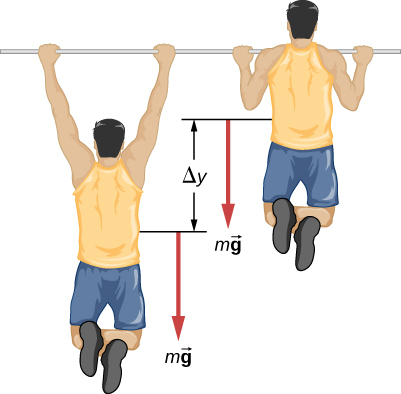Strategy
The work done against gravity, going up or down a distance $\Delta y$, is $mg\text{Δ}y.$ (If you lift and lower yourself at constant speed, the force you exert cancels gravity over the whole pull-up cycle.) Thus, the work done by the trainee’s muscles (moving, but not accelerating, his body) for a complete repetition (up and down) is $2mg\text{Δ}y.$ Let’s assume that $\text{Δ}y=2\text{ft}\approx 60\phantom{\rule{0.2em}{0ex}}\text{cm}\text{.}$ Also, assume that the arms comprise 10% of the body mass and are not included in the moving mass. With these assumptions, we can calculate the work done for 10 pull-ups and divide by 10 s to get the average power.

Solution
The result we get, applying our assumptions, is

${P}_{\text{ave}}=\frac{10\phantom{\rule{0.2em}{0ex}}×\phantom{\rule{0.2em}{0ex}}2\left(0.9\phantom{\rule{0.2em}{0ex}}×\phantom{\rule{0.2em}{0ex}}80\phantom{\rule{0.2em}{0ex}}\text{kg}\right)\left(9.8\phantom{\rule{0.2em}{0ex}}{\text{m/s}}^{2}\right)\left(0.6\phantom{\rule{0.2em}{0ex}}\text{m}\right)}{10\phantom{\rule{0.2em}{0ex}}\text{s}}=850\phantom{\rule{0.2em}{0ex}}\text{W}\text{.}$

Significance
This is typical for power expenditure in strenuous exercise; in everyday units, it’s somewhat more than one horsepower $\left(1\phantom{\rule{0.2em}{0ex}}\text{hp}=746\phantom{\rule{0.2em}{0ex}}\text{W}\right).$

Check Your Understanding Estimate the power expended by a weightlifter raising a 150-kg barbell 2 m in 3 s.

980 W

The power involved in moving a body can also be expressed in terms of the forces acting on it. If a force $\stackrel{\to }{F}$ acts on a body that is displaced $d\stackrel{\to }{r}$ in a time dt, the power expended by the force is

$P=\frac{dW}{dt}=\frac{\stackrel{\to }{F}·d\stackrel{\to }{r}}{dt}=\stackrel{\to }{F}·\left(\frac{d\stackrel{\to }{r}}{dt}\right)=\stackrel{\to }{F}·\stackrel{\to }{v},$

where $\stackrel{\to }{v}$ is the velocity of the body. The fact that the limits implied by the derivatives exist, for the motion of a real body, justifies the rearrangement of the infinitesimals.

Automotive Power Driving Uphill
How much power must an automobile engine expend to move a 1200-kg car up a 15% grade at 90 km/h ((Figure))? Assume that 25% of this power is dissipated overcoming air resistance and friction.

We want to calculate the power needed to move a car up a hill at constant speed.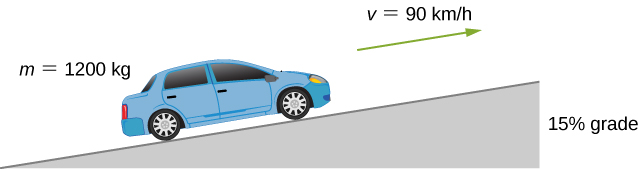Strategy
At constant velocity, there is no change in kinetic energy, so the net work done to move the car is zero. Therefore the power supplied by the engine to move the car equals the power expended against gravity and air resistance. By assumption, 75% of the power is supplied against gravity, which equals $m\stackrel{\to }{g}·\stackrel{\to }{v}=mgv\phantom{\rule{0.2em}{0ex}}\text{sin}\phantom{\rule{0.2em}{0ex}}\theta ,$ where $\theta$ is the angle of the incline. A 15% grade means $\text{tan}\phantom{\rule{0.2em}{0ex}}\theta =0.15.$ This reasoning allows us to solve for the power required.

Solution
Carrying out the suggested steps, we find

$0.75\phantom{\rule{0.2em}{0ex}}P=mgv\phantom{\rule{0.2em}{0ex}}\text{sin}\left({\text{tan}}^{-1}\phantom{\rule{0.2em}{0ex}}0.15\right),$

or

$P=\frac{\left(1200\phantom{\rule{0.2em}{0ex}}×\phantom{\rule{0.2em}{0ex}}9.8\phantom{\rule{0.2em}{0ex}}\text{N}\right)\left(90\phantom{\rule{0.2em}{0ex}}\text{m}\text{/}3.6\phantom{\rule{0.2em}{0ex}}\text{s}\right)\text{sin}\left(8.53\text{°}\right)}{0.75}=58\phantom{\rule{0.2em}{0ex}}\text{kW,}$

or about 78 hp. (You should supply the steps used to convert units.)

Significance
This is a reasonable amount of power for the engine of a small to mid-size car to supply $\left(1\phantom{\rule{0.2em}{0ex}}\text{hp}=0.746\phantom{\rule{0.2em}{0ex}}\text{kW}\text{).}$ Note that this is only the power expended to move the car. Much of the engine’s power goes elsewhere, for example, into waste heat. That’s why cars need radiators. Any remaining power could be used for acceleration, or to operate the car’s accessories.

### Summary

• Power is the rate of doing work; that is, the derivative of work with respect to time.
• Alternatively, the work done, during a time interval, is the integral of the power supplied over the time interval.
• The power delivered by a force, acting on a moving particle, is the dot product of the force and the particle’s velocity.

### Key Equations

 Work done by a force over an infinitesimal displacement $dW=\stackrel{\to }{F}·d\stackrel{\to }{r}=|\stackrel{\to }{F}||d\stackrel{\to }{r}|\text{cos}\phantom{\rule{0.2em}{0ex}}\theta$ Work done by a force acting along a path from A to B ${W}_{AB}=\underset{\text{path}AB}{\int }\stackrel{\to }{F}·d\stackrel{\to }{r}$ Work done by a constant force of kinetic friction ${W}_{\text{fr}}=\text{−}{f}_{k}|{l}_{AB}|$ Work done going from A to B by Earth’s gravity, near its surface ${W}_{\text{grav,}AB}=\text{−}mg\left({y}_{B}-{y}_{A}\right)$ Work done going from A to B by one-dimensional spring force ${W}_{\text{spring,}AB}=\text{−}\left(\frac{1}{2}k\right)\left({x}_{B}^{2}-{x}_{A}^{2}\right)$ Kinetic energy of a non-relativistic particle $K=\frac{1}{2}m{v}^{2}=\frac{{p}^{2}}{2m}$ Work-energy theorem ${W}_{\text{net}}={K}_{B}-{K}_{A}$ Power as rate of doing work $P=\frac{dW}{dt}$ Power as the dot product of force and velocity $P=\stackrel{\to }{F}·\stackrel{\to }{v}$

### Conceptual Questions

Most electrical appliances are rated in watts. Does this rating depend on how long the appliance is on? (When off, it is a zero-watt device.) Explain in terms of the definition of power.

Appliances are rated in terms of the energy consumed in a relatively small time interval. It does not matter how long the appliance is on, only the rate of change of energy per unit time.

Explain, in terms of the definition of power, why energy consumption is sometimes listed in kilowatt-hours rather than joules. What is the relationship between these two energy units?

A spark of static electricity, such as that you might receive from a doorknob on a cold dry day, may carry a few hundred watts of power. Explain why you are not injured by such a spark.

The spark occurs over a relatively short time span, thereby delivering a very low amount of energy to your body.

Does the work done in lifting an object depend on how fast it is lifted? Does the power expended depend on how fast it is lifted?

Can the power expended by a force be negative?

If the force is antiparallel or points in an opposite direction to the velocity, the power expended can be negative.

How can a 50-W light bulb use more energy than a 1000-W oven?

### Problems

A person in good physical condition can put out 100 W of useful power for several hours at a stretch, perhaps by pedaling a mechanism that drives an electric generator. Neglecting any problems of generator efficiency and practical considerations such as resting time: (a) How many people would it take to run a 4.00-kW electric clothes dryer? (b) How many people would it take to replace a large electric power plant that generates 800 MW?

a. 40; b. 8 million

What is the cost of operating a 3.00-W electric clock for a year if the cost of electricity is ?0.0900 per $\text{kW}·\text{h}$?

A large household air conditioner may consume 15.0 kW of power. What is the cost of operating this air conditioner 3.00 h per day for 30.0 d if the cost of electricity is ?0.110 per $\text{kW}·\text{h}$?

?149

(a) What is the average power consumption in watts of an appliance that uses 5.00 $\text{kW}·\text{h}$ of energy per day? (b) How many joules of energy does this appliance consume in a year?

(a) What is the average useful power output of a person who does $6.00\phantom{\rule{0.2em}{0ex}}×\phantom{\rule{0.2em}{0ex}}{10}^{6}\phantom{\rule{0.2em}{0ex}}\text{J}$ of useful work in 8.00 h? (b) Working at this rate, how long will it take this person to lift 2000 kg of bricks 1.50 m to a platform? (Work done to lift his body can be omitted because it is not considered useful output here.)

a. 208 W; b. 141 s

A 500-kg dragster accelerates from rest to a final speed of 110 m/s in 400 m (about a quarter of a mile) and encounters an average frictional force of 1200 N. What is its average power output in watts and horsepower if this takes 7.30 s?

(a) How long will it take an 850-kg car with a useful power output of 40.0 hp (1 hp equals 746 W) to reach a speed of 15.0 m/s, neglecting friction? (b) How long will this acceleration take if the car also climbs a 3.00-m high hill in the process?

a. 3.20 s; b. 4.04 s

(a) Find the useful power output of an elevator motor that lifts a 2500-kg load a height of 35.0 m in 12.0 s, if it also increases the speed from rest to 4.00 m/s. Note that the total mass of the counterbalanced system is 10,000 kg—so that only 2500 kg is raised in height, but the full 10,000 kg is accelerated. (b) What does it cost, if electricity is ?0.0900 per $\text{kW}·\text{h}$
?

(a) How long would it take a $1.50\phantom{\rule{0.2em}{0ex}}\phantom{\rule{0.2em}{0ex}}×\phantom{\rule{0.2em}{0ex}}{10}^{5}\text{-kg}$ airplane with engines that produce 100 MW of power to reach a speed of 250 m/s and an altitude of 12.0 km if air resistance were negligible? (b) If it actually takes 900 s, what is the power? (c) Given this power, what is the average force of air resistance if the airplane takes 1200 s? (Hint: You must find the distance the plane travels in 1200 s assuming constant acceleration.)

a. 224 s; b. 24.8 MW; c. 49.7 kN

Calculate the power output needed for a 950-kg car to climb a $2.00\text{°}$ slope at a constant 30.0 m/s while encountering wind resistance and friction totaling 600 N.

A man of mass 80 kg runs up a flight of stairs 20 m high in 10 s. (a) how much power is used to lift the man? (b) If the man’s body is 25% efficient, how much power does he expend?

a. 1.57 kW; b. 6.28 kW

The man of the preceding problem consumes approximately $1.05\phantom{\rule{0.2em}{0ex}}×\phantom{\rule{0.2em}{0ex}}{10}^{7}\phantom{\rule{0.2em}{0ex}}\text{J}$ (2500 food calories) of energy per day in maintaining a constant weight. What is the average power he produces over a day? Compare this with his power production when he runs up the stairs.

An electron in a television tube is accelerated uniformly from rest to a speed of $8.4\phantom{\rule{0.2em}{0ex}}×\phantom{\rule{0.2em}{0ex}}{10}^{7}\phantom{\rule{0.2em}{0ex}}\text{m/s}$ over a distance of 2.5 cm. What is the power delivered to the electron at the instant that its displacement is 1.0 cm?

$6.83\text{μW}$

Coal is lifted out of a mine a vertical distance of 50 m by an engine that supplies 500 W to a conveyer belt. How much coal per minute can be brought to the surface? Ignore the effects of friction.

A girl pulls her 15-kg wagon along a flat sidewalk by applying a 10-N force at $37\text{°}$ to the horizontal. Assume that friction is negligible and that the wagon starts from rest. (a) How much work does the girl do on the wagon in the first 2.0 s. (b) How much instantaneous power does she exert at $t=2.0\phantom{\rule{0.2em}{0ex}}\text{s}$?

a. 8.51 J; b. 8.51 W

A typical automobile engine has an efficiency of 25%. Suppose that the engine of a 1000-kg automobile has a maximum power output of 140 hp. What is the maximum grade that the automobile can climb at 50 km/h if the frictional retarding force on it is 300 N?

When jogging at 13 km/h on a level surface, a 70-kg man uses energy at a rate of approximately 850 W. Using the facts that the “human engine” is approximately 25% efficient, determine the rate at which this man uses energy when jogging up a $5.0\text{°}$ slope at this same speed. Assume that the frictional retarding force is the same in both cases.

1.7 kW

A cart is pulled a distance D on a flat, horizontal surface by a constant force F that acts at an angle $\theta$ with the horizontal direction. The other forces on the object during this time are gravity (${F}_{w}$), normal forces (${F}_{N1}$) and (${F}_{N2}$), and rolling frictions ${F}_{r1}$ and ${F}_{r2}$, as shown below. What is the work done by each force?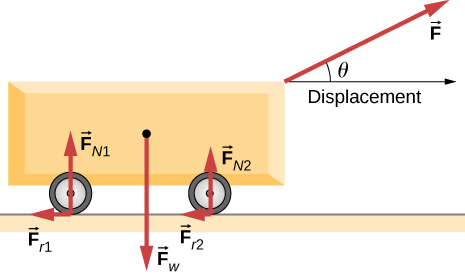Consider a particle on which several forces act, one of which is known to be constant in time: ${\stackrel{\to }{F}}_{1}=\left(3\phantom{\rule{0.2em}{0ex}}\text{N}\right)\stackrel{^}{i}+\left(4\phantom{\rule{0.2em}{0ex}}\text{N}\right)\stackrel{^}{j}.$ As a result, the particle moves along the x-axis from $x=0$ to $x=5\phantom{\rule{0.2em}{0ex}}\text{m}$ in some time interval. What is the work done by ${\stackrel{\to }{F}}_{1}$
?

$15\phantom{\rule{0.2em}{0ex}}\text{N}·\text{m}$

Consider a particle on which several forces act, one of which is known to be constant in time: ${\stackrel{\to }{F}}_{1}=\left(3\phantom{\rule{0.2em}{0ex}}\text{N}\right)\stackrel{^}{i}+\left(4\phantom{\rule{0.2em}{0ex}}\text{N}\right)\stackrel{^}{j}.$ As a result, the particle moves first along the x-axis from $x=0$ to $x=5\phantom{\rule{0.2em}{0ex}}\text{m}$ and then parallel to the y-axis from $y=0$ to $y=6\phantom{\rule{0.2em}{0ex}}\text{m}\text{.}$ What is the work done by ${\stackrel{\to }{F}}_{1}$
?

Consider a particle on which several forces act, one of which is known to be constant in time: ${\stackrel{\to }{F}}_{1}=\left(3\phantom{\rule{0.2em}{0ex}}\text{N}\right)\stackrel{^}{i}+\left(4\phantom{\rule{0.2em}{0ex}}\text{N}\right)\stackrel{^}{j}.$ As a result, the particle moves along a straight path from a Cartesian coordinate of (0 m, 0 m) to (5 m, 6 m). What is the work done by ${\stackrel{\to }{F}}_{1}$
?

$39\phantom{\rule{0.2em}{0ex}}\text{N}·\text{m}$

Consider a particle on which a force acts that depends on the position of the particle. This force is given by ${\stackrel{\to }{F}}_{1}=\left(2y\right)\stackrel{^}{i}+\left(3x\right)\stackrel{^}{j}.$ Find the work done by this force when the particle moves from the origin to a point 5 meters to the right on the x-axis.

A boy pulls a 5-kg cart with a 20-N force at an angle of $30\text{°}$ above the horizontal for a length of time. Over this time frame, the cart moves a distance of 12 m on the horizontal floor. (a) Find the work done on the cart by the boy. (b) What will be the work done by the boy if he pulled with the same force horizontally instead of at an angle of $30\text{°}$ above the horizontal over the same distance?

a. $208\phantom{\rule{0.2em}{0ex}}\text{N}·\text{m}$; b. $240\phantom{\rule{0.2em}{0ex}}\text{N}·\text{m}$

A crate of mass 200 kg is to be brought from a site on the ground floor to a third floor apartment. The workers know that they can either use the elevator first, then slide it along the third floor to the apartment, or first slide the crate to another location marked C below, and then take the elevator to the third floor and slide it on the third floor a shorter distance. The trouble is that the third floor is very rough compared to the ground floor. Given that the coefficient of kinetic friction between the crate and the ground floor is 0.100 and between the crate and the third floor surface is 0.300, find the work needed by the workers for each path shown from A to E. Assume that the force the workers need to do is just enough to slide the crate at constant velocity (zero acceleration). Note: The work by the elevator against the force of gravity is not done by the workers.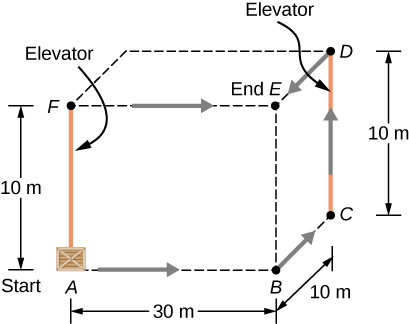A hockey puck of mass 0.17 kg is shot across a rough floor with the roughness different at different places, which can be described by a position-dependent coefficient of kinetic friction. For a puck moving along the x-axis, the coefficient of kinetic friction is the following function of x, where x is in m: $\mu \left(x\right)=0.1+0.05x.$ Find the work done by the kinetic frictional force on the hockey puck when it has moved (a) from $x=0$ to $x=2\phantom{\rule{0.2em}{0ex}}\text{m}$, and (b) from $x=2\phantom{\rule{0.2em}{0ex}}\text{m}$ to $x=4\phantom{\rule{0.2em}{0ex}}\text{m}$.

a. $\text{−}0.9\phantom{\rule{0.2em}{0ex}}\text{N}·\text{m}$; b. $-0.83\phantom{\rule{0.2em}{0ex}}\text{N}·\text{m}$

A horizontal force of 20 N is required to keep a 5.0 kg box traveling at a constant speed up a frictionless incline for a vertical height change of 3.0 m. (a) What is the work done by gravity during this change in height? (b) What is the work done by the normal force? (c) What is the work done by the horizontal force?

A 7.0-kg box slides along a horizontal frictionless floor at 1.7 m/s and collides with a relatively massless spring that compresses 23 cm before the box comes to a stop. (a) How much kinetic energy does the box have before it collides with the spring? (b) Calculate the work done by the spring. (c) Determine the spring constant of the spring.

a. 10. J; b. 10. J; c. 380 N/m

You are driving your car on a straight road with a coefficient of friction between the tires and the road of 0.55. A large piece of debris falls in front of your view and you immediate slam on the brakes, leaving a skid mark of 30.5 m (100-feet) long before coming to a stop. A policeman sees your car stopped on the road, looks at the skid mark, and gives you a ticket for traveling over the 13.4 m/s (30 mph) speed limit. Should you fight the speeding ticket in court?

A crate is being pushed across a rough floor surface. If no force is applied on the crate, the crate will slow down and come to a stop. If the crate of mass 50 kg moving at speed 8 m/s comes to rest in 10 seconds, what is the rate at which the frictional force on the crate takes energy away from the crate?

160 J/s

Suppose a horizontal force of 20 N is required to maintain a speed of 8 m/s of a 50 kg crate. (a) What is the power of this force? (b) Note that the acceleration of the crate is zero despite the fact that 20 N force acts on the crate horizontally. What happens to the energy given to the crate as a result of the work done by this 20 N force?

Grains from a hopper falls at a rate of 10 kg/s vertically onto a conveyor belt that is moving horizontally at a constant speed of 2 m/s. (a) What force is needed to keep the conveyor belt moving at the constant velocity? (b) What is the minimum power of the motor driving the conveyor belt?

a. 10 N; b. 20 W

A cyclist in a race must climb a $5\text{°}$ hill at a speed of 8 m/s. If the mass of the bike and the biker together is 80 kg, what must be the power output of the biker to achieve the goal?

### Challenge Problems

Shown below is a 40-kg crate that is pushed at constant velocity a distance 8.0 m along a $30\text{°}$ incline by the horizontal force $\stackrel{\to }{F}.$ The coefficient of kinetic friction between the crate and the incline is ${\mu }_{k}=0.40.$ Calculate the work done by (a) the applied force, (b) the frictional force, (c) the gravitational force, and (d) the net force.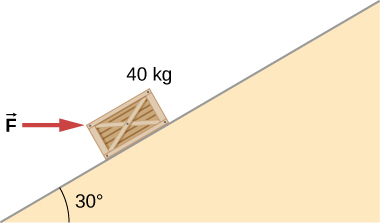If crate goes up: a. 3.46 kJ; b. −1.89 kJ; c. −1.57 kJ; d. 0; If crate goes down: a. −0.39 kJ; b. −1.18 kJ; c. 1.57 kJ; d. 0

The surface of the preceding problem is modified so that the coefficient of kinetic friction is decreased. The same horizontal force is applied to the crate, and after being pushed 8.0 m, its speed is 5.0 m/s. How much work is now done by the force of friction? Assume that the crate starts at rest.

The force F(x) varies with position, as shown below. Find the work done by this force on a particle as it moves from $x=1.0\phantom{\rule{0.2em}{0ex}}\text{m}$ to $x=5.0\phantom{\rule{0.2em}{0ex}}\text{m}\text{.}$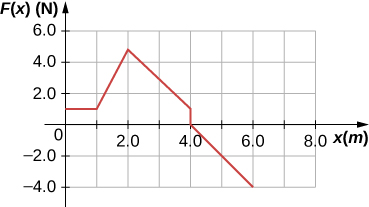8.0 J

Find the work done by the same force in (Figure), between the same points, $A=\left(0,0\right)\phantom{\rule{0.2em}{0ex}}\text{and}\phantom{\rule{0.2em}{0ex}}B=\left(2\phantom{\rule{0.2em}{0ex}}\text{m},2\phantom{\rule{0.2em}{0ex}}\text{m}\right)$, over a circular arc of radius 2 m, centered at (0, 2 m). Evaluate the path integral using Cartesian coordinates. (Hint: You will probably need to consult a table of integrals.)

Answer the preceding problem using polar coordinates.

35.7 J

Find the work done by the same force in (Figure), between the same points, $A=\left(0,0\right)\phantom{\rule{0.2em}{0ex}}\text{and}\phantom{\rule{0.2em}{0ex}}B=\left(2\phantom{\rule{0.2em}{0ex}}\text{m},2\phantom{\rule{0.2em}{0ex}}\text{m}\right)$, over a circular arc of radius 2 m, centered at (2 m, 0). Evaluate the path integral using Cartesian coordinates. (Hint: You will probably need to consult a table of integrals.)

Answer the preceding problem using polar coordinates.

24.3 J

Constant power P is delivered to a car of mass m by its engine. Show that if air resistance can be ignored, the distance covered in a time t by the car, starting from rest, is given by $s={\left(8P\text{/}9m\right)}^{1\text{/}2}{t}^{3\text{/}2}.$

Suppose that the air resistance a car encounters is independent of its speed. When the car travels at 15 m/s, its engine delivers 20 hp to its wheels. (a) What is the power delivered to the wheels when the car travels at 30 m/s? (b) How much energy does the car use in covering 10 km at 15 m/s? At 30 m/s? Assume that the engine is 25% efficient. (c) Answer the same questions if the force of air resistance is proportional to the speed of the automobile. (d) What do these results, plus your experience with gasoline consumption, tell you about air resistance?

a. 40 hp; b. 39.8 MJ, independent of speed; c. 80 hp, 79.6 MJ at 30 m/s; d. If air resistance is proportional to speed, the car gets about 22 mpg at 34 mph and half that at twice the speed, closer to actual driving experience.

Consider a linear spring, as in (Figure)(a), with mass M uniformly distributed along its length. The left end of the spring is fixed, but the right end, at the equilibrium position $x=0,$ is moving with speed v in the x-direction. What is the total kinetic energy of the spring? (Hint: First express the kinetic energy of an infinitesimal element of the spring dm in terms of the total mass, equilibrium length, speed of the right-hand end, and position along the spring; then integrate.)

### Glossary

average power
work done in a time interval divided by the time interval
power
(or instantaneous power) rate of doing work